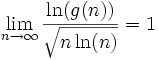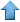Precision RecruitingData Mining Contest Math Jobs * Site Map
 [ Home ] [ Finance ] [ Web Audit ] [ Consulting ]

These are difficult mathematical questions. They are arising from real applications such as fraud detection, arbitrage and scoring systems. If you have interesting answers to any questions, feel free to email us your comments or solution. The best answers will be published here. Companies and Organizations interested in submitting problems should E-mail us.

Permutations of maximum period

Problem:

Let p be a permutation on [n] = (1,...,n). We are considering operations on the permutation group of order n. Let us define p2 as p o p, and more generally pk+1 as p o pk. For instance, if n=3 and p = (3,1,2), then p2 = (2,3,1) is obtained by applying p twice, and p3 = (1,2,3) = I. We shall use the letter I to denote the identity permutation: I = (1,2,...,n). Then the period is the minimum integer k > 0 such that pk = I.

For a given n, we are interested in finding permutations with maximum period. Let us note that the permutation (2,3,4,...,n-1,n,1) always has a period of n. As a consequence, the following permutation with n=12 with 3 cycles (sub permutations) defined as p=(2,3,1|5,6,7,4|9,10,11,12,8) has a period 3 x 4 x 5 = 60. This is true because the three sub-periods - 3, 4 and 5 - are mutually prime. In that case the period is the product of the sub-periods. More generally, if we had m cycles, the period could be as large as (n/m)m. This expression attains its maximum when m = 0.368 x n (0.368 = 1/e). In other words, the maximum is achieved when we have about m/3 cycles with mutually prime periods, regardless of n. The maximum period is then of the order en/e < n!

Is my reasoning correct? I am trying to create random permutations to encrypt a string s, in the context of fraud detection. The idea is that permutations with large periods, if well chosen, will be very difficult to identify. Let's say that p has an even period v. Then if you only know pv/2 but not p nor v, it is almost impossible to identify p and v. Once a hacker knows p and v, he can easly retrieve the original string s.

Contributions:

• From David Bernier:

This is related to Landau's function g(n) , n being the number of elements in the set getting permuted.• From Derek Holt:

I have a C program to identify permutations of maximum order. For example, for n=10000, the maximum order is

317*313*311*307*293*283*281*277*271*269*263*257*251*241*239*233*229*227* 223*211*199*197*193*191*181*179*173*167*163*157*151*149*139*137*131*127* 113*109*107*103*101*97*89*83*79*73*71*67*61*59*53*47*43*41*37*31*29*23* 19*17*169*121*49*25*81*64 =

837248314781153836222627527908638519798608214039954646068895157070382622 91218453286312511300740953193197240510692638318426636506681182400Data Mining • Machine Learning • Analytics • Quant • Statistics • Econometrics • Biostatistics • Web Analytics • Business Intelligence • Risk Management • Operations Research • AI • Predictive Modeling • Actuarial Sciences • Statistical Programming • Customer Insight • Data Modeling • Competitive Intelligence • Market Research • Information Retrieval • Computer Science • Retail Analytics • Healthcare Analytics • ROI Optimization • Design Of Experiments • Scoring Models • Six Sigma • SAS • Splus • SAP • ETL • SPSS • CRM • Cloud Computing • Electrical Engineering • Fraud Detection • Marketing Databases • Data Analysis • Decision Science • Text Mining